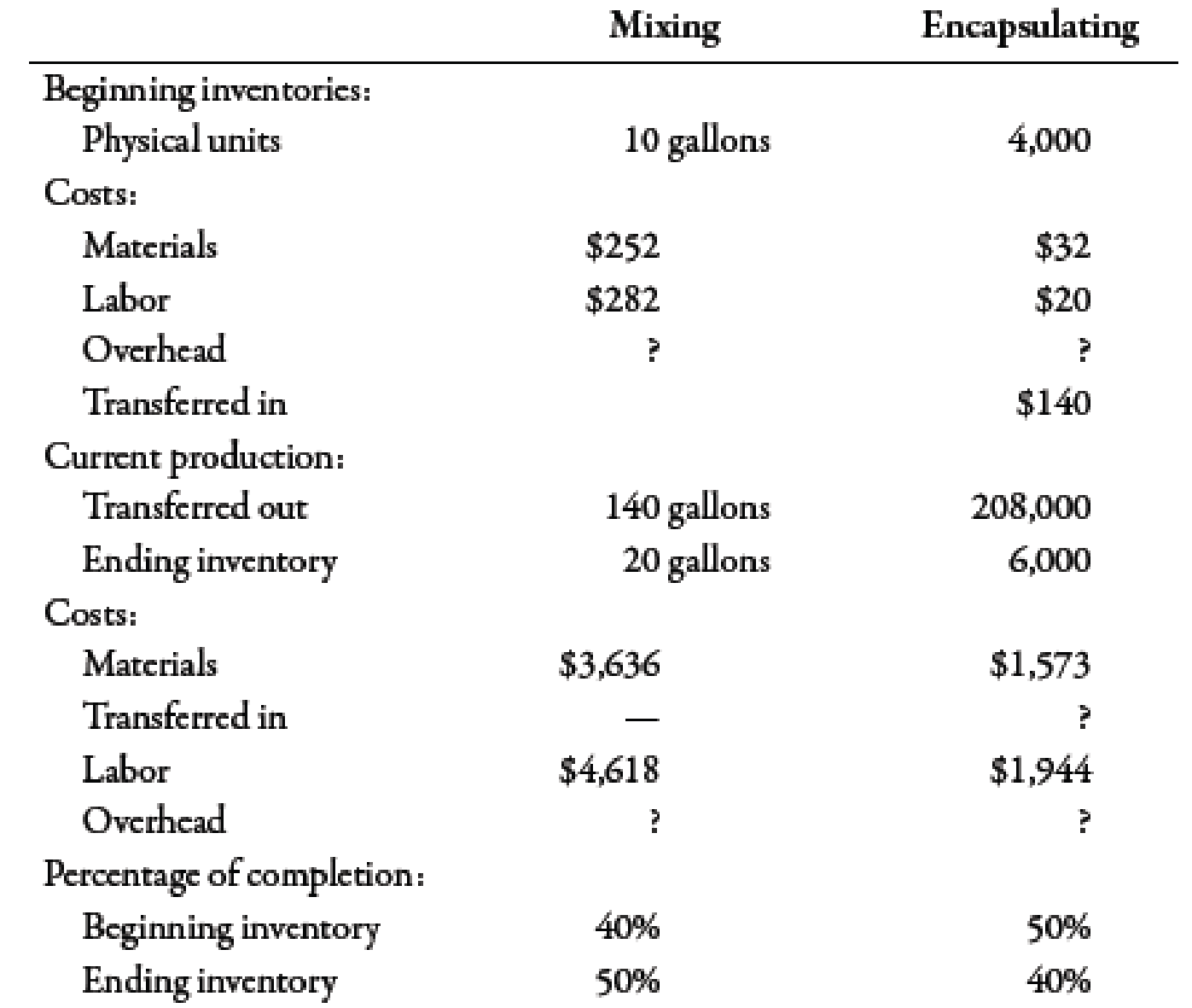# Benson Pharmaceuticals uses a process-costing system to compute the unit costs of the over-the-counter cold remedies that it produces. It has three departments: mixing, encapsulating, and bottling. In mixing, the ingredients for the cold capsules are measured, sifted, and blended (with materials assumed to be uniformly added throughout the process). The mix is transferred out in gallon containers. The encapsulating department takes the powdered mix and places it in capsules (which are necessarily added at the beginning of the process). One gallon of powdered mix converts into 1,500 capsules. After the capsules are filled and polished, they are transferred to bottling, where they are placed in bottles that are then affixed with a safety seal, lid, and label. Each bottle receives 50 capsules. During March, the following results are available for the first two departments: Overhead in both departments is applied as a percentage of direct labor costs. In the mixing department, overhead is 200% of direct labor. In the encapsulating department, the overhead rate is 150% of direct labor. Required: 1. Prepare a production report for the mixing department using the weighted average method. Follow the five steps outlined in the chapter. ( Note : Round to two decimal places for the unit cost.) 2. Prepare a production report for the encapsulating department using the weighted average method. Follow the five steps outlined in the chapter. ( Note : Round to four decimal places for the unit cost.) 3. CONCEPTUAL CONNECTION Explain why the weighted average method is easier to use than FIFO. Explain when weighted average will give about the same results as FIFO.### Managerial Accounting: The Corners...

7th Edition
Maryanne M. Mowen + 2 others
Publisher: Cengage Learning
ISBN: 9781337115773### Managerial Accounting: The Corners...

7th Edition
Maryanne M. Mowen + 2 others
Publisher: Cengage Learning
ISBN: 9781337115773

#### Solutions

Chapter
Section
Chapter 6, Problem 67P
Textbook Problem
1169 views

## Benson Pharmaceuticals uses a process-costing system to compute the unit costs of the over-the-counter cold remedies that it produces. It has three departments: mixing, encapsulating, and bottling. In mixing, the ingredients for the cold capsules are measured, sifted, and blended (with materials assumed to be uniformly added throughout the process). The mix is transferred out in gallon containers. The encapsulating department takes the powdered mix and places it in capsules (which are necessarily added at the beginning of the process). One gallon of powdered mix converts into 1,500 capsules. After the capsules are filled and polished, they are transferred to bottling, where they are placed in bottles that are then affixed with a safety seal, lid, and label. Each bottle receives 50 capsules.During March, the following results are available for the first two departments:Overhead in both departments is applied as a percentage of direct labor costs. In the mixing department, overhead is 200% of direct labor. In the encapsulating department, the overhead rate is 150% of direct labor.Required: 1. Prepare a production report for the mixing department using the weighted average method. Follow the five steps outlined in the chapter. (Note: Round to two decimal places for the unit cost.) 2. Prepare a production report for the encapsulating department using the weighted average method. Follow the five steps outlined in the chapter. (Note: Round to four decimal places for the unit cost.) 3. CONCEPTUAL CONNECTION Explain why the weighted average method is easier to use than FIFO. Explain when weighted average will give about the same results as FIFO.

Expert Solution

1.

To determine

Present a production report for the mixing department using weighted average method.

### Explanation of Solution

Weighted Average Method:

Weighted average method is an inventory valuation method. In this method, cost is divided by equivalent units to obtain unit cost. This unit cost is used to value the inventory units.

Step 1: Physical flow analysis:

 Particulars Units Units to account for: Units in beginning WIP 10 Add: Units started during the period1 150 Units to account for 160 Units accounted for: Units completed and transferred 140 Add: Units in ending WIP 20 Units accounted for 160

Table (1)

Step 2: Computation of equivalent units:

 Particulars Units Units completed and transferred 140 Equivalent units from ending inventory (20×50%) 10 Equivalent units 150

Table (2)

Step 3: Computation of unit cost:

 Particulars Amount ($) Cost of beginning inventory: Material 252 Labor 282 Overhead (200%×$282) 564 Total cost of beginning inventory (A) 1,098 Cost incurred: Material 3,636 Labor 4,618 Overhead (200%×$4,618) 9,236 Total cost incurred (B) 17,490 Total manufacturing cost (A+B) 18,588 Unit cost (Total manufacturing costEquivalent units) ($18,588150) 123
Expert Solution

2.

To determine

Present a production report for the encapsulating department using weighted average method.

Expert Solution

3.

To determine

Discuss whether or not weighted average method is easier than FIFO. Also, discuss the situation in which weighted average will give about the same results as FIFO.

### Want to see the full answer?

Check out a sample textbook solution.See solution

### Want to see this answer and more?

Bartleby provides explanations to thousands of textbook problems written by our experts, many with advanced degrees!

See solution